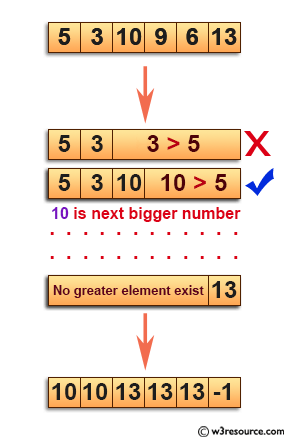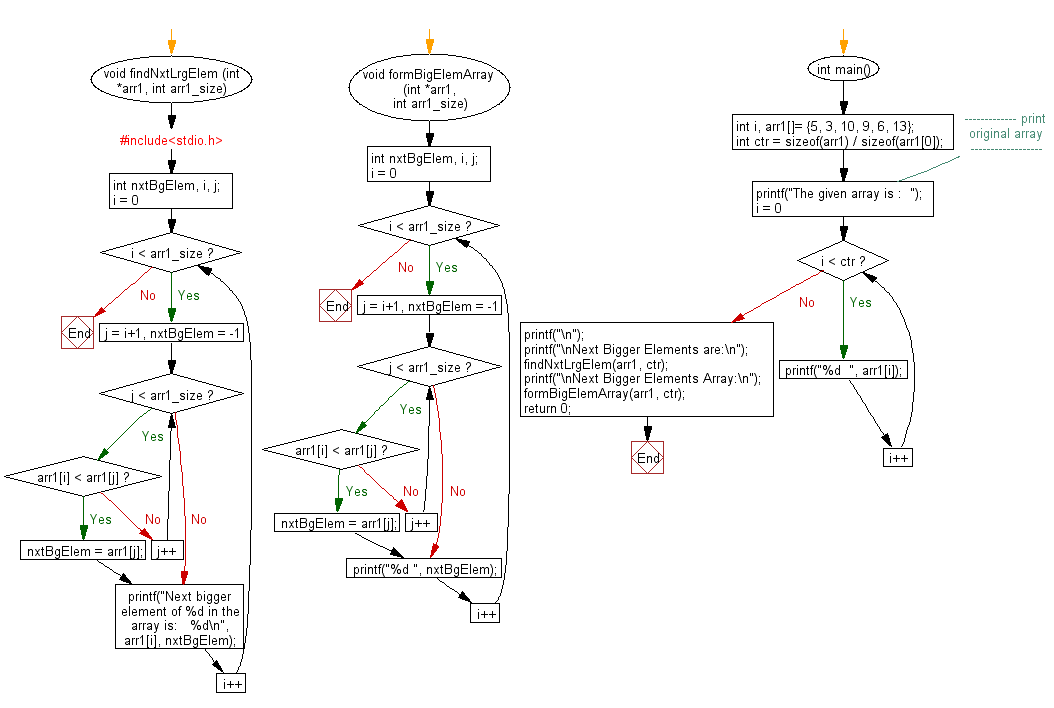﻿ C exercises: Find the next greater elements in a given unsorted array - w3resource# C Exercises: Find the next greater elements in a given unsorted array

## C Array: Exercise-43 with Solution

Write a program in C to to print next greater elements in a given unsorted array. Elements for which no greater element exist, consider next greater element as -1.

Pictorial Presentation:Sample Solution:

C Code:

``````#include <stdio.h>

void findNxtLrgElem (int *arr1, int arr1_size)
{
int nxtBgElem, i, j;
for(i = 0; i < arr1_size; i++)
{
for (j = i+1, nxtBgElem = -1; j < arr1_size; j++)
{
if (arr1[i] < arr1[j])
{
nxtBgElem = arr1[j];
break;
}
}
printf("Next bigger element of %d in the array is:   %d\n", arr1[i], nxtBgElem);
}
}
void formBigElemArray (int *arr1, int arr1_size)
{
int nxtBgElem, i, j;
for(i = 0; i < arr1_size; i++)
{
for (j = i+1, nxtBgElem = -1; j < arr1_size; j++)
{
if (arr1[i] < arr1[j])
{
nxtBgElem = arr1[j];
break;
}
}
printf("%d ", nxtBgElem);
}
}
int main()
{
int i, arr1[]= {5, 3, 10, 9, 6, 13};
int ctr = sizeof(arr1) / sizeof(arr1);
//------------- print original array ------------------
printf("The given array is :  ");
for(i = 0; i < ctr; i++)
{
printf("%d  ", arr1[i]);
}
printf("\n");
//-----------------------------------------------------
printf("\nNext Bigger Elements are:\n");
findNxtLrgElem(arr1, ctr);

printf("\nNext Bigger Elements Array:\n");
formBigElemArray(arr1, ctr);
return 0;
}
```
```

Sample Output:

```The given array is :  5  3  10  9  6  13

Next Bigger Elements are:
Next bigger element of 5 in the array is:   10
Next bigger element of 3 in the array is:   10
Next bigger element of 10 in the array is:   13
Next bigger element of 9 in the array is:   13
Next bigger element of 6 in the array is:   13
Next bigger element of 13 in the array is:   -1

Next Bigger Elements Array:
10 10 13 13 13 -1
```

Flowchart:C Programming Code Editor:

Improve this sample solution and post your code through Disqus.

What is the difficulty level of this exercise?

﻿